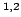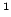Statistica Sinica 18(2008), 425-442

LARGE SAMPLE COVARIANCE MATRICES WITHOUT

INDEPENDENCE STRUCTURES IN COLUMNS

Zhidong Baiand Wang ZhouNortheast Normal University andNational University of Singapore

Abstract: The limiting spectral distribution of large sample covariance matrices is derived under dependence conditions. As applications, we obtain the limiting spectral distributions of Spearman's rank correlation matrices, sample correlation matrices, sample covariance matrices from finite populations, and sample covariance matrices from causal AR(1) models.

Key words and phrases: AR(1) model, finite population, limiting spectral distribution, random matrix theory, sample correlation matrices, sample covariance matrices, Spearman's rank correlation matrices.